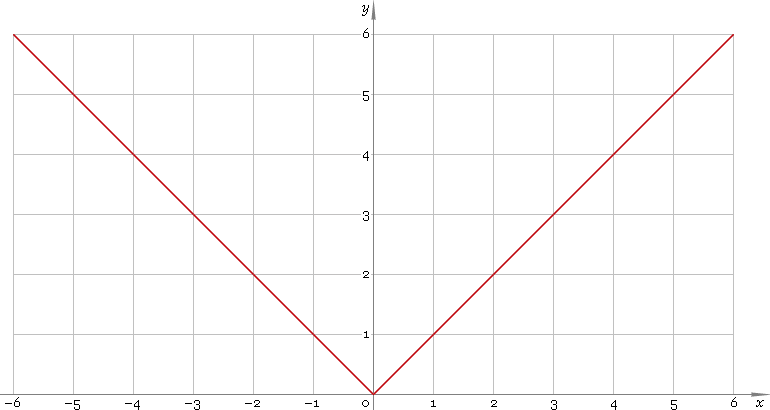The Art of Mathematics

# abs — absolute value function

## 1. Definition

Absolute value function is defined as

|x| = x for x ≥ 0;
|x| = −x for x < 0.

## 2. Graph

Absolute value function is defined everywhere on real axis. Its graph is depicted below — fig. 1.Fig. 1. Graph of the absolute value function y = |x|.

Function codomain is non-negative half of the real axis: [0, +∞).

## 3. Identities

Function is symmetrical:

|−x| = |x|

Sum and difference of arguments:

|x + y| = |x| + |y|, if signx = signy
|x + y| = ||x| − |y||, if signx ≠ signy
|xy| = ||x| − |y||, if signx = signy
|xy| = |x| + |y|, if signx ≠ signy

Product and ratio of arguments:

|xy| = |x||y|
|x /y| = |x| /|y|

## 4. Derivative and indefinite integral

Absolute value derivative:

|x|′ = 1 for x > 0;
|x|′ = −1 for x < 0.

For 0 the derivative is undefined.

Indefinite integral of the absolute value:

∫ |x| dx = signx x2/2 + C

where sign is a signum function and C is an arbitrary constant.

## 5. How to use

To get absolute value of the number:

``abs(−1);``

To calculate absolute value of the complex number:

``abs(−1+i);``

To get absolute value of the current result:

``abs(rslt);``

To get absolute value of the number z in calculator memory:

``abs(mem[z]);``

## 6. Support

Absolute value of the real number is supported in free version of the Librow calculator.

Absolute value of the complex number is supported in professional version of the Librow calculator.